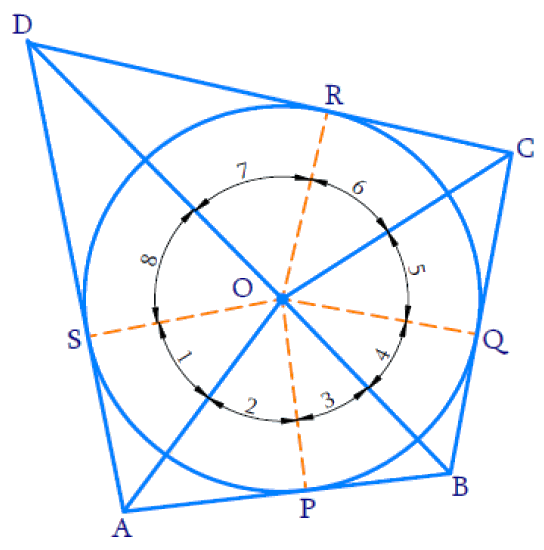# Ex.10.2 Q13 Circles Solution - NCERT Maths Class 10

Go back to  'Ex.10.2'

## Question

Prove that opposite sides of a quadrilateral circumscribing a circle subtend supplementary angles at the center of the circle.

Video Solution
Circles
Ex 10.2 | Question 13

## Text Solution

To prove:

Opposite sides of a quadrilateral circumscribing a circle subtend supplementary angles at the center of the circle.

Steps and Reasoning:We know that, tangents drawn from an external point to a circle subtend equal angles at the center.

In the above figure, $${P,\;Q,\;R,\;S}$$ are point of contacts

$${AS = AP}$$ (The lengths of tangents drawn from an external point $$A$$ to a circle are equal.)

\begin{align} \angle {SOA} = \angle {POA} = \angle 1 = \angle 2 \end{align}

Tangents drawn from an external point to a circle subtend equal angles at the centre.

Similarly,

\begin{align} \angle 3 = \angle 4 , \angle 5 = \angle 6 , \angle 7 = \angle 8 \end{align}

Since complete angle is $${360^ \circ }$$,

\begin{align}\left[ \begin{array} & \angle 1+\angle 2+\angle 3+\angle 4+ \\ \angle 5+\angle 6+\angle 7+\angle 8 \\ \end{array} \right]&={{360}^{^\circ }} \\2(\angle 1+\angle 8+\angle 4+\angle 5)&={{360}^{^\circ }} \\ \,\angle 1+\angle 8+\angle 4+\angle 5&={{180}^{{}^\circ }} \\ \end{align}

[or]

\begin{align} 2(\angle 2+\angle 3+\angle 6+\angle 7) &=360^{\circ} \\ \angle 2+\angle 3+\angle 6+\angle 7 &=180^{\circ} \\ \angle A O B+\angle C O D &=180^{\circ} \end{align}

From above figure \begin{align}\angle A O D+\angle B O C =180^{\circ}\end{align}

$$\angle {AOD}$$ and $$\angle {BOC}$$ are angles subtended by opposite sides of quadrilateral circumscribing a circle and sum of them is $${180^ \circ }$$

Hence proved.

Learn from the best math teachers and top your exams

• Live one on one classroom and doubt clearing
• Practice worksheets in and after class for conceptual clarity
• Personalized curriculum to keep up with school